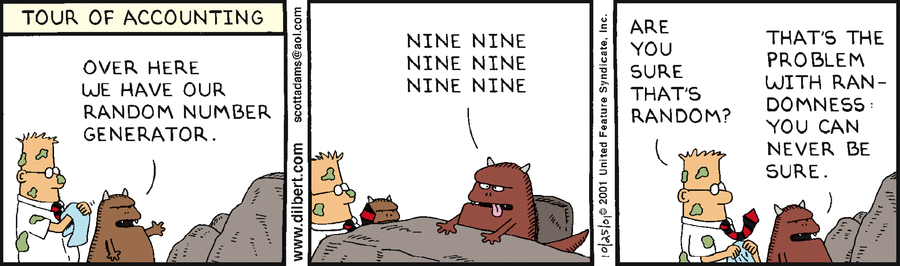# Number - Random Weighted (Weighted dice)

How to generate random data that is not normal (With a Given Distribution) ?

For instance, how to generate data for a weighted dice (also known as weighted or crooked die) with the following weights:

• Number 1: 1
• Number 2: 3
• Number 3: 1
• Number 4: 2
• Number 5: 1
• Number 6: 4

## Algorithm

### Weighted list

• Make a weighted list:
{ 1, 2, 2, 2, 3, 4, 4, 5, 6, 6, 6, 6 }

• Generate the list index randomly in order to get your element in the list. The list index is then a random number that is 0 or greater and is less than the length of the list (ie sum of the weights)

$\text{random list index} = (\text{random number between 0 and 1}) * 12$

• Pick your element in the list corresponding to this random index

### Sum of weights

• Calculate the sum of all the weights:

$1 + 3 + 1 + 2 + 1 + 4 = 12$

• Pick a random number that is 0 or greater and is less than the sum of the weights
• Go through the items one at a time, subtracting their weight from your random number, until you get the item where the random number is less than that item's weight

## Documentation / Reference

Discover MoreNumber - Random (Stochastic|Independent) or (Balanced)

Think of randomness as a lack of pattern. Something random should be unpredictable. We shouldn’t be able to predict the next value of the sequence The degree to which a system has no pattern is known...